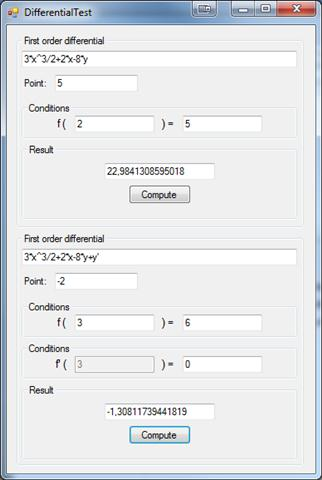### Features And Algorithms

This library uses numerical calculator library to resolve differential equations so can handle same functions.

Mathematical formulas used to resolve differential equations are Runge–Kutta methods. Default step used in computation is 0.001.

### Example

Take function f(x)=3x^3+2x^2

It derivatives are:
f'(x)=9*x^2+x
f''(x)=18*x

Let us assume that we only know second derivative f''(x) formula and that f'(0)=2 and f(0)=0.
It's enough informations to compute f(x) function value at any point. Example how to compute f(x) value at 2 using this library is below.

### Usage

First order derivative:
`string formula = "9*x^2+2";double lookingPoint = 2;double startingPoint = 0;double startingPointFunctionValue = 0;Differential diff = new Differential(formula);double resultFirstOrder = diff.ComputeDifferential(lookingPoint, startingPoint, startingPointFunctionValue);`

Second order derivative:
`string formula = "18*x";double lookingPoint = 2;double startingPoint = 0;double startingPointFunctionValue = 0;double startingPointFunctionValueII = 2;Differential diff = new Differential(formula);double resultFirstOrder = diff.ComputeDifferentialII(lookingPoint, startingPoint,                                                     startingPointFunctionValue, startingPointFunctionValueII);`

### Example application